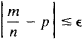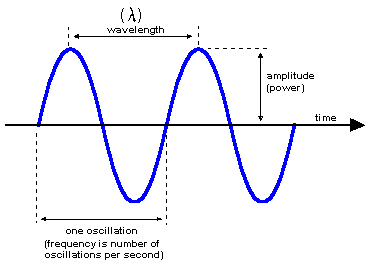# frequency

(redirected from Frequency (wave motion))
Also found in: Dictionary, Thesaurus, Medical.

## frequency

frequency: see harmonic motion; wave.

## Frequency (wave motion)

The number of times which sound pressure, electrical intensity, or other quantities specifying a wave vary from their equilibrium value through a complete cycle in unit time. The most common unit of frequency is the hertz (Hz), which is equal to 1 cycle per second. In one cycle there is a positive variation from equilibrium, a return to equilibrium, then a negative variation, and return to equilibrium. This relationship is often described in terms of the sine wave, and the frequency referred to is that of an equivalent sine-wave variation in the parameter under discussion. See Frequency measurement, Sine wave, Wave motion

McGraw-Hill Concise Encyclopedia of Physics. © 2002 by The McGraw-Hill Companies, Inc.

## frequency

Symbol: f , ν. The number of oscillations per unit time of a vibrating system. Frequency is measured in hertz. The frequency of a wave is the number of wave crests passing a point per unit time. For light and other electromagnetic radiation, it is related to wavelength λ by ν = c /λ, where c is the speed of light.
Collins Dictionary of Astronomy © Market House Books Ltd, 2006
The following article is from The Great Soviet Encyclopedia (1979). It might be outdated or ideologically biased.

## Frequency

The frequency of an event A is the ratio m/n of the number m of occurrences of A in a given series of trials to the total number n of trials. If the trials are independent and there is a definite probability p of the occurrence of A in an individual trial, then, for arbitrarily small ∊ > 0, at sufficiently large m it is practically certain that the frequency m/n satisfies the inequality(seeLARGE NUMBERS, LAW OF and PROBABILITY).

The term “frequency” is used in mathematical statistics to designate the number of elements of a set that have a specified attribute.

The Great Soviet Encyclopedia, 3rd Edition (1970-1979). © 2010 The Gale Group, Inc. All rights reserved.

## frequency

[′frē·kwən·sē]
(physics)
The number of cycles completed by a periodic quantity in a unit time.
(statistics)
The number of times an event or item falls into or is expected to fall into a certain class or category.
McGraw-Hill Dictionary of Scientific & Technical Terms, 6E, Copyright © 2003 by The McGraw-Hill Companies, Inc.

## frequency

The number of oscillations per second (a) of the current or voltage in an alternating-current electric circuit, or (b) of a sound wave, or (c) of a vibrating solid object; expressed in hertz (abbr. Hz) or in cycles per second (abbr. cps).
McGraw-Hill Dictionary of Architecture and Construction. Copyright © 2003 by McGraw-Hill Companies, Inc.

## frequency

i. The number of recurrences of a periodic phenomenon in a unit of time.
ii. The number of cycles completed in one second. One cycle per second is the basic unit of measurement of frequency and is called a hertz.
iii. The number of services operated by an airline per day or per week over a particular route.

## frequency

1. Physics the number of times that a periodic function or vibration repeats itself in a specified time, often 1 second. It is usually measured in hertz.
2. Ecology
a. the number of individuals of a species within a given area
b. the percentage of quadrats that contains individuals of a species
Collins Discovery Encyclopedia, 1st edition © HarperCollins Publishers 2005

## frequency

The number of oscillations (vibrations) in one second. Frequency is measured in Hertz (Hz), which is the same as "oscillations per second" or "cycles per second." For example, the alternating current in a wall outlet in the U.S. and Canada is 60Hz. Electromagnetic radiation is measured in kiloHertz (kHz), megahertz (MHz) and gigahertz (GHz). See wavelength, frequency response, audio, carrier and space/time.

FrequencyThe frequency is the number of oscillations per second. The higher the frequency (the closer the ripples would be in this diagram) and the shorter the wavelength.
Copyright © 1981-2019 by The Computer Language Company Inc. All Rights reserved. THIS DEFINITION IS FOR PERSONAL USE ONLY. All other reproduction is strictly prohibited without permission from the publisher.
Site: Follow: Share:
Open / Close